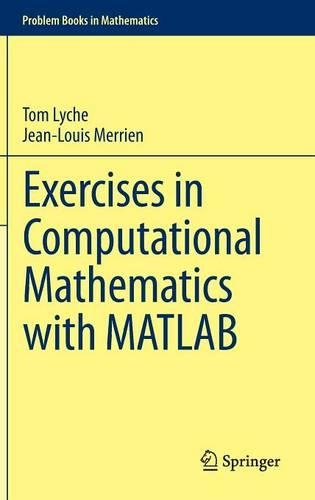•# Exercises in Computational Mathematics with MATLAB - Problem Books in Mathematics (Hardback)

(author), (author)
£24.99
Hardback 372 Pages / Published: 16/09/2014
• Click & Collect only

Only available in some of our shops

This product is currently unavailable.

Designed to provide tools for independent study, this book contains student-tested mathematical exercises joined with MATLAB programming exercises.

Most chapters open with a review followed by theoretical and programming exercises, with detailed solutions provided for all problems including programs. Many of the MATLAB exercises are presented as Russian dolls: each question improves and completes the previous program and results are provided to validate the intermediate programs.

The book offers useful MATLAB commands, advice on tables, vectors, matrices and basic commands for plotting. It contains material on eigenvalues and eigenvectors and important norms of vectors and matrices including perturbation theory; iterative methods for solving nonlinear and linear equations; polynomial and piecewise polynomial interpolation; Bezier curves; approximations of functions and integrals and more. The last two chapters considers ordinary differential equations including two point boundary value problems, and deal with finite difference methods for some partial differential equations.

The format is designed to assist students working alone, with concise Review paragraphs, Math Hint footnotes on the mathematical aspects of a problem and MATLAB Hint footnotes with tips on programming.

Publisher: Springer-Verlag Berlin and Heidelberg GmbH & Co. KG
ISBN: 9783662435106
Number of pages: 372
Weight: 6978 g
Dimensions: 235 x 155 x 22 mm

MEDIA REVIEWS

From the book reviews:

"This is a very interesting and useful book for any advanced undergraduate and beginning graduate student on mathematics, statistics, computational physics, chemistry, and engineering, with a focus on numerical analysis and computational science. The main scope of this book is to provide students with the opportunity to apply numerical analysis and the well-known MATLAB to solve problems in their own specialties." (T. E. Simos, Computing Reviews, January, 2015)

"This is an interesting new kind of book in the area of numerical analysis. ... It is widely accepted that solving exercises is essential to achieve a deeper understanding of a mathematical topic. Under this point of view the present book can be seen as an adequate vehicle to really get into the field of numerical analysis. ... the book can also serve as a rich source of exercises for university courses." (Rolf Dieter Grigorieff, zbMATH, Vol. 1304, 2015)